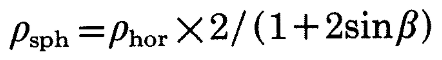# Implementation of Reflection Angular Dependence

Hello all,

Problem Statement
Am I implementing an equation for canopy reflection angular dependence accurately?

Equation
A validated formula to estimate the angular dependence of canopy reflection is as follows:where rho_hor (ρ_hor) represents a constant reflection and absorption property of the leaves and beta (β) represents the angle between a ray and the horizontal surface of a canopy.

Implementation
To implement this equation, I created a .cal file for use with a BRTD function. The BRTDfunc and cal file are:

=== BRTD start ===

void BRTDfunc canopy_ref
10 refl_red refl_grn refl_blu 0 0 0 0 0 0 BRTDcanopy.cal
0
9 0 0 0 0 0 0 0 0 0

=== BRTD end ===

=== .cal file start ===

{
canopy_reflection.cal
}

horizontalsurface = sqrt(NxP * NxP + NyP * NyP);
ray = sqrt(bottom + NzP * NzP);
sinB = horizontalsurface / ray;

refl_red = 0.044 * (2 / (1 + 2 * sinB));
refl_grn = 0.067 * (2 / (1 + 2 * sinB));
refl_blu = 0.027 * (2 / (1 + 2 * sinB));

=== file end ===

While the Radiance engine accepts these BRTDfunc and .cal file inputs, I am skeptical of my implementation.

This suspicion emerged because I have not seen any examples of a person implementing angular dependence using NxP, NyP, and NzP. Unfortunately, my queries through the discourse forums keep returning examples discussing “solid angle” concepts, a different concept from the one I am exploring.